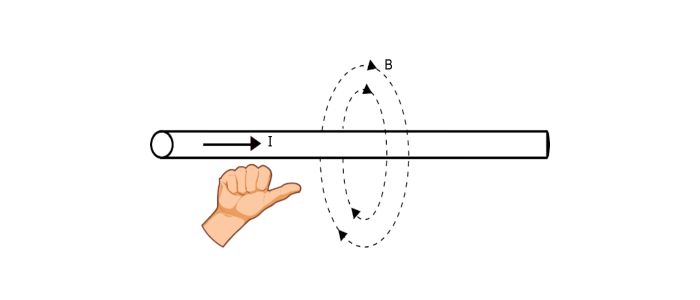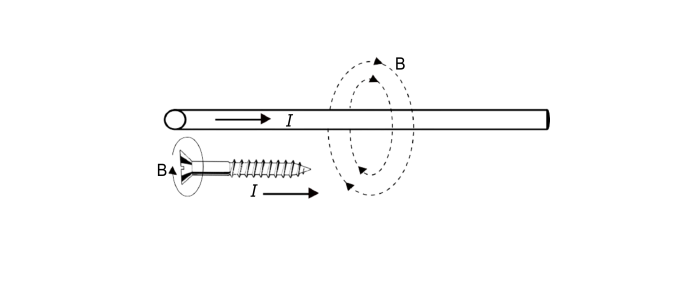# Magnetic Field around a Current Carrying Conductor

The space or field in which a magnetic pole experiences a force is called as a magnetic field. The magnetic field can be produced either by moving the charge or some magnetic material. During the beginning of 19th century, a scientist named H. C. Oersted discovered that when current flows through a conductor, a magnetic field produces around it.

## Magnetic Field around a Current Carrying Conductor

As the current is defined as the rate of flow of electric charge. According to electromagnetic field theory, a moving charge produces a magnetic field which is proportional to the current, thus a carrying conductor produces magnetic field around it. The magnetic field has both magnitude and direction, hence it a vector quantity and denoted by B. The strength of magnetic field due to current carrying conductor depends on the amount of current in the conductor and distance of the point from the conductor.

When an electric current flows through a conductor, a magnetic field is set up all along the length of the conductor. The magnetic field lines around the conductor are in the form of concentric circles. The direction of magnetic field lines depends upon the direction of current.

The direction of magnetic field around the current carrying conductor can be determined by −

• Right Hand Rule

• Cork Screw Rule

## Right Hand Rule

This rule states that, hold the conductor in right hand with the thumb pointing in the direction of current. Then the curled fingers will point in the direction of magnetic field around the conductor (As shown in the figure).## Cork Screw Rule

According to this rule, hold the cork screw in the right hand and rotate it in such a way that it moves in the direction of current. Then the direction in which the hand rotates will be the direction of magnetic field lines around the conductor.## Strength of Magnetic Field due to a Current Carrying Conductor

Magnetic Field Strength is given by,

$$H=\frac{NI}{l}\:AT/m$$

Where, 𝑁 = number of turns = 1,

𝑙 = Distance of point from the conductor, and

Magnetic Flux Density, $$B=\mu_{0}H$$

## Properties of Magnetic Field Produced by Current Carrying Conductor

• The strength of magnetic field is directly proportional to the magnitude of current.

• The strength of magnetic field at any point is inversely proportional to the distance of the point from the conductor.

• The magnetic field encircles the conductor.

• The magnetic field lies in a plane perpendicular to the conductor.

• The magnetic field can be reversed by reversing the direction of current in the conductor.

Updated on: 29-May-2021

2K+ Views